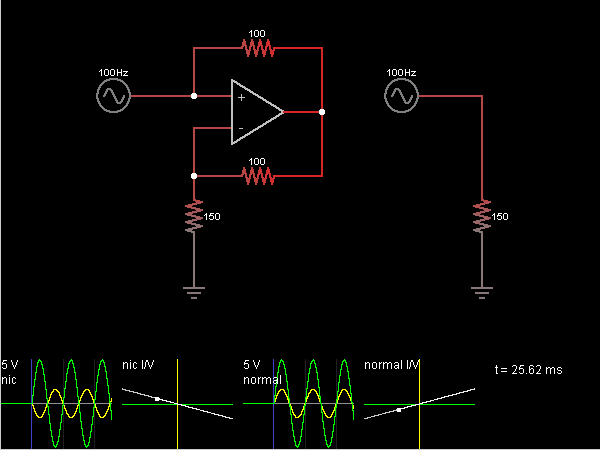# Circuit Simulator - Negative Impedance Converter

Negative Impedance ConverterCircuit Description:
The circuit on the left converts a positive impedance to a negative impedance. So, for example, instead of Ohm's Law (E=IR) it causes a resistor to obey E=-IR. The circuit on the right shows a positive impedance (a 150 ohm resistor) for comparison.

The op-amp attempts to keep its – input at the same voltage as the + input, which is connected to the input signal. So the impedance being transformed (a 150 ohm resistor) responds as if it were connected directly to the input signal. Whatever current it needs is sourced by the op-amp and flows through the bottom 100 ohm resistor.

Since the + input is at the same voltage as the – input, the current and voltage drop across the top 100 ohm resistor must be the same as the bottom one. As a result, when the input voltage is positive, current is flowing into the input rather than out of it. The input current is the same as the current through the impedance, but in the opposite direction.

Discussion:
Be the first person to comment on this question !